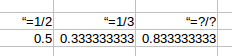# Fractions in Python

When you ask your spreadsheet to calculate 1/2 + 1/3 you get something like this:This is obviously an approximation. The 3’s after the decimal point repeat indefinitely.

• 1/2 = 3/6
• 1/3 = 2/6
• 1/2 + 1/3 = 3/6 + 2/6 = 5/6

Python is a simple but powerful language, and comes with a wealth of libraries. Its Fractions library gives you the correct answer in a couple of lines

Here is the annotated code. You can find the raw code at the GitHub repository

1. from fractions import Fraction